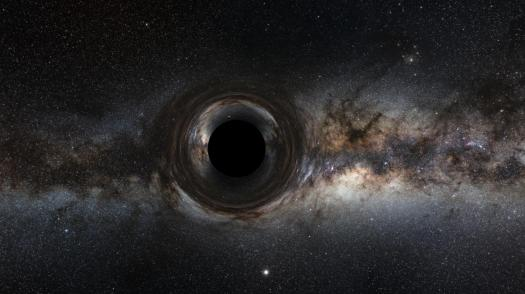# Black Hole: Trivia Quiz Questions!

10 Questions | Total Attempts: 225SettingsHave you ever asked, “What happens if the density of matter in a region becomes so high that not even light can escape? ”, Einstein once asked though. Black hole is exactly described by that question. Don't even think black hole is an empty space, No it's not, it is a great amount of matter packed into a very small area. It is important to note that most black holes are formed from the remnants of a large star that dies in a supernova explosion. Can you top this quiz? Give it a try.

• 1.
How many layers can be found in a black hole?
• A.

9

• B.

7

• C.

1

• D.

3

• 2.
Which of these is true about black holes?
• A.

It's has angular Velocity

• B.

Black Hole emits light

• C.

Two black holes can not meet

• D.

It has strong gravity

• 3.
What is bound to happen in places where gravity is heavy?
• A.

Heavy fall

• B.

Future travel

• C.

Space escape

• D.

Neutralisation

• 4.
When a black hole achieves a stable condition after formation, which of these does it not posses?
• A.

Angular momentum

• B.

Mass

• C.

Charge

• D.

Thorque

• 5.
Which of these does a Schwarzschild black holes has?
• A.

Angular momentum

• B.

Mass

• C.

Charge

• D.

Thorque

• 6.
Which of these will destroy a black hole?
• A.

Merging of black holes

• B.

Multiple explosion

• C.

Water poured into a black hole

• D.

• 7.
Whose theorem states that the simplest black hole is the only vacuum solution that is vertically symmetric?
• A.

Bowman

• B.

Einstein

• C.

Birkholf

• D.

Rutherford

• 8.
Into how many are black holes classified?
• A.

8

• B.

7

• C.

4

• D.

9

• 9.
Which year was  the first modern solution of general relativity that would characterize a black hole found?
• A.

1916

• B.

1986

• C.

2001

• D.

180BC

• 10.
Which of these descriptions about photon Spheres is true?
• A.

Zero thickness

• B.

56mm thick

• C.

Round shape

• D.

Fluidity

Related TopicsBack to top